• 设定绘图坐标的FontSize属性，这种设定同时对坐标轴标注、图例、标题有效 h = gca; set (h, 'FontSize' ,msize);% 设置文字大小，同时影响坐标轴标注、图例、标题等 % x= 0 : 0.2 : 12 ; % plot(x,sin(x), '-' ,x,...
% Plot the image of the Sensitivity and False Positive per image
clear;
close all;
clc;
I1 =[0.5 75];
I2 =[1 54.5];

msize =19; %设定字体的大小
hold on
plot(I1(1),I1(2),'ko-','MarkerFaceColor','r')  % 红色实心圆点
text(I1(1)+0.1,I1(2),'Wei 2002')
hold on
plot(I2(1),I2(2),'ko-','MarkerFaceColor','g')  % 绿色实心圆点
text(I2(1)+0.1,I2(2),'Carreira 1998','fontsize',msize)
hold on

legend('Wei 2002','Carreira 1998')
axis on;
axis tight
grid on
axis([0 8 0 100]);
ylabel('Sensitivity')
xlabel('False Positivies per image')

% 用set设定绘图坐标的FontSize属性，这种设定同时对坐标轴标注、图例、标题有效
h = gca;
set(h,'FontSize',msize);% 设置文字大小，同时影响坐标轴标注、图例、标题等

% x=0:0.2:12;
%       plot(x,sin(x),'-',x,1.5*cos(x),':')
%       legend('First','Second',-1);    %强行将注释视窗放在图形视窗的外右边。

效果图如下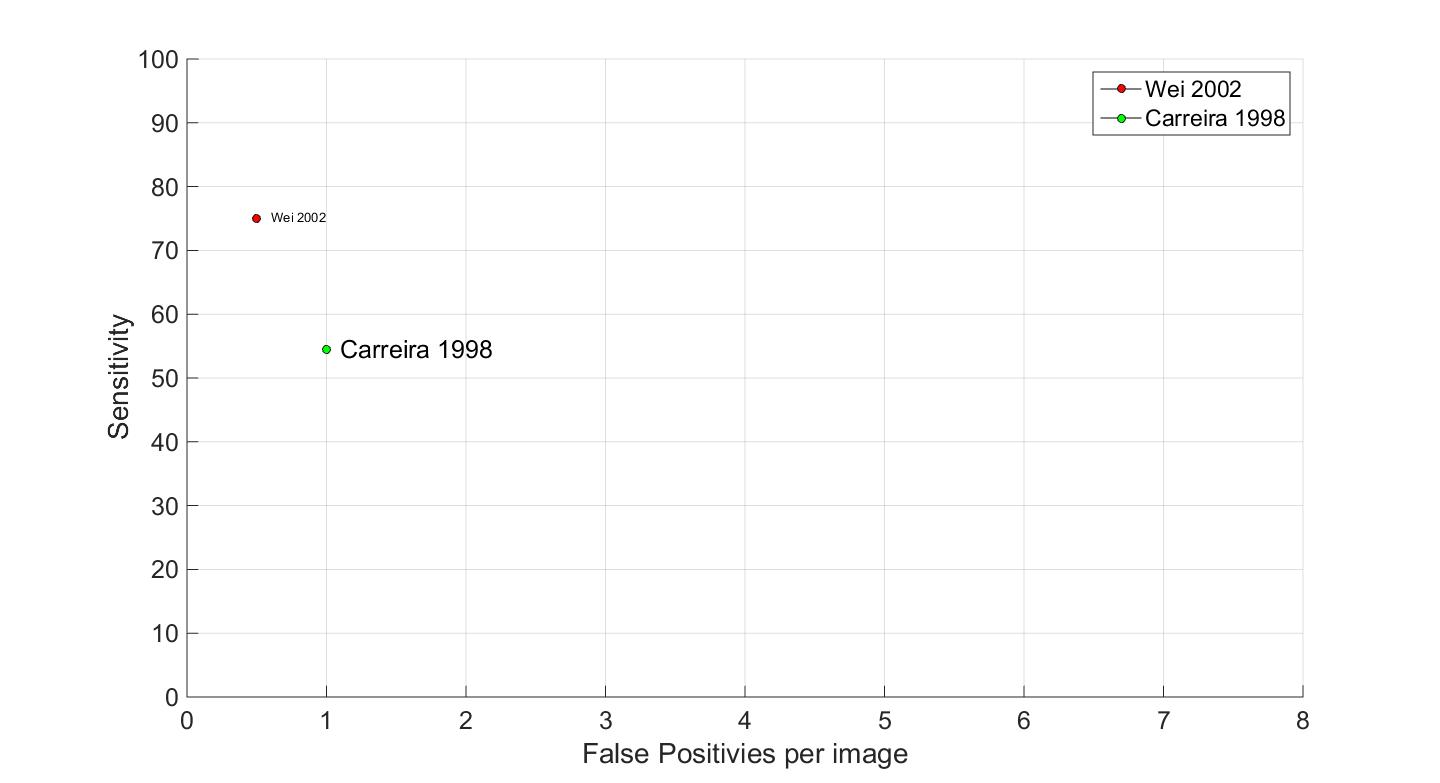展开全文• 目录 ...text函数是向数据点添加文本说明。 一.语法 text(x,y,txt) text(x,y,z,txt) text(___,Name,Value) text(ax,___) t = text(___) 1.输入参数 x-第一个坐标 第一个坐标，..
目录
一.语法
1.输入参数
2名称-值对组参数
3.输出参数
二.说明
三.示例
1.向数据点添加文本说明
2.向多个数据点添加文本
3.显示多行文本
4.指定文本大小和颜色
5.修改现有文本

text函数是向数据点添加文本说明。
一.语法
text(x,y,txt)

text(x,y,z,txt)

text(___,Name,Value)

text(ax,___)

t = text(___)
1.输入参数

x - 第一个坐标

第一个坐标，指定为下列形式之一：
标量 - 将文本添加到单个点。  向量 - 将文本添加到多个点。
第一个坐标的解释取决于坐标区的类型：
对于笛卡尔坐标区，第一个坐标是以数据单位表示的 x 轴位置。要更改单位，请设置 Text 对象的Units属性。  对于极坐标区，第一个坐标是以弧度表示的极坐标角 θ。  对于地理坐标区，第一个坐标是纬度（以度为单位）。
x 和 y 的大小必须相同。
若要控制相对于指定点的文本放置，可以使用 Text 对象的HorizontalAlignment、VerticalAlignment 和 Extent 属性。
数据类型： double | categorical | datetime | duration

y - 第二个坐标

第二个坐标，指定为下列形式之一：
标量 - 将文本添加到单个点。  向量 - 将文本添加到多个点。
第二个坐标的解释取决于坐标区的类型：
对于笛卡尔坐标区，第二个坐标是以数据单位表示的 y 轴位置。要更改单位，请设置 Text 对象的Units属性。  对于极坐标区，第二个坐标是以数据单位表示的半径。  对于地理坐标区，第二个坐标是经度（以度为单位）。
x 和 y 的大小必须相同。
若要控制相对于指定点的文本放置，可以使用使用 Text 对象的HorizontalAlignment、VerticalAlignment 和 Extent 属性。
数据类型： double | categorical | datetime | duration

z - 第三个坐标

第三个坐标，指定为下列形式之一：
标量 - 将文本添加到单个点。  向量 - 将文本添加到多个点。
第三个坐标的解释取决于坐标区的类型：
对于笛卡尔坐标区，第三个坐标是以数据单位表示的 z 轴位置。要更改单位，请设置 Text 对象的Units属性。  对于极坐标区和地理坐标区，第三个坐标会影响坐标区中文本的分层。
x、y 和 z 的大小必须相同。
若要控制相对于指定点的文本放置，可以使用 Text 对象的HorizontalAlignment、VerticalAlignment 和 Extent 属性。
数据类型： double | categorical | datetime | duration

txt - 要显示的文本

要显示的文本，指定为字符向量、字符串标量、字符数组、字符串数组、元胞数组或分类数组。
用于单个数据点的文本
要为单个点添加文本，请将 txt 指定为字符向量或字符串标量。例如，text(.5,.5,'my text')。
对于多行文本，请：
使用元胞数组，其中每个元胞包含一行文本。例如，text(.5,.5,{'first','second'})。  使用字符串数组，其中每个元素是一行文本。例如，text(.5,.5,["first","second"])。  使用具有多个行的字符数组，其中每一行包含相同数量的字符。例如，text(.5,.5,['first '; 'second'])。  使用 sprintf 创建一个换行符。例如，text(.5,.5,sprintf('first \nsecond'))。
如果将文本指定为分类数组，MATLAB® 将使用数组中的值，而不是类别。
用于多个数据点的文本
要在每个位置显示相同的文本，请将 txt 指定为字符向量或字符串。例如，text([0 1],[0 1],'my text')。
要在每个位置显示不同文本，请使用元胞数组。例如，text([0 1],[0 1],{'first','second'})。
对于多行文本，请使用嵌套元胞数组。例如，text([0 1],[0 1],{{'first','new line'},'second'})。
希腊字母、特殊字符和数值变量
要包括特殊字符（例如上标、下标、希腊字母或数学符号），请使用 TeX 标记。有关支持的标记的列表，参考Interpreter 属性。
要在文本中包含数值变量，可以使用num2str函数。
v = 42;
txt = ['The value is ',num2str(v)];

ax - 目标坐标区

目标坐标区，指定为 Axes 对象、PolarAxes 对象或 GeographicAxes 对象。如果不指定坐标区，则 text 使用当前坐标区。
2名称-值对组参数
指定可选的、以逗号分隔的 Name,Value 对组参数。Name 为参数名称，Value 为对应的值。Name 必须放在引号中。可采用任意顺序指定多个名称-值对组参数，如 Name1,Value1,...,NameN,ValueN 所示。
例如：text(.5,.5,'my text','FontSize',14,'Color','red')

注意

此处所列的属性只是一部分。有关完整列表，请参考Text属性。

'FontSize' - 字体大小

字体大小，指定为大于 0 的标量值（以磅为单位）。默认字体大小取决于具体操作系统和区域设置。一磅等于 1/72 英寸。要更改字体单位，请使用 FontUnits 属性。
例如： 12
数据类型： single | double | int8 | int16 | int32 | int64 | uint8 | uint16 | uint32 | uint64

'FontWeight' - 字符粗细

字符粗细，指定为 'normal' 或 'bold'。MATLAB 使用 FontWeight 属性从系统提供的字体中选择一种字体。并非所有字体都有加粗字体。因此，指定加粗字体仍可能得到普通字体。

'FontName' - 字体名称

字体名称，指定为支持的字体名称或 'FixedWidth'。要正确显示和打印文本，必须选择系统支持的字体。默认字体取决于操作系统和区域设置。
要使用在任何区域设置中都有较好显示效果的等宽字体，请使用 'FixedWidth'。等宽字体依赖于根FixedWidthFontName属性。设置根 FixedWidthFontName 属性会导致立即更新显示方式以使用新字体。

'Color' - 文本颜色

文本颜色，指定为 RGB 三元组、十六进制颜色代码、颜色名称或短名称。默认值 [0 0 0] 对应于黑色。对于自定义颜色，请指定 RGB 三元组或十六进制颜色代码。
RGB 三元组是包含三个元素的行向量，其元素分别指定颜色中红、绿、蓝分量的强度。强度值必须位于 [0,1] 范围内，例如 [0.4 0.6 0.7]。  十六进制颜色代码是字符向量或字符串标量，以井号 (#) 开头，后跟三个或六个十六进制数字，范围可以是 0 到 F。这些值不区分大小写。因此，颜色代码 '#FF8800' 与 '#ff8800'、'#F80' 与 '#f80' 是等效的。
此外，还可以按名称指定一些常见的颜色。下表列出了命名颜色选项、等效 RGB 三元组和十六进制颜色代码。
颜色名称短名称RGB 三元组十六进制颜色代码外观'red''r'[1 0 0]'#FF0000''green''g'[0 1 0]'#00FF00''blue''b'[0 0 1]'#0000FF''cyan''c'[0 1 1]'#00FFFF''magenta''m'[1 0 1]'#FF00FF''yellow''y'[1 1 0]'#FFFF00''black''k'[0 0 0]'#000000''white''w'[1 1 1]'#FFFFFF''none'不适用不适用不适用无颜色
以下是 MATLAB 在许多类型的绘图中使用的默认颜色的 RGB 三元组和十六进制颜色代码。
RGB 三元组十六进制颜色代码外观[0 0.4470 0.7410]'#0072BD'[0.8500 0.3250 0.0980]'#D95319'[0.9290 0.6940 0.1250]'#EDB120'[0.4940 0.1840 0.5560]'#7E2F8E'[0.4660 0.6740 0.1880]'#77AC30'[0.3010 0.7450 0.9330]'#4DBEEE'[0.6350 0.0780 0.1840]'#A2142F'例如： 'blue'     [0 0 1]    '#0000FF'

'HorizontalAlignment' - 相对于位置点水平对齐文本

相对于 Position 属性中的 x 值水平对齐文本，指定为此表中的一个值。垂直线指示 x 值相对于文本的位置。
值结果'left'（默认值）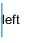'center'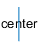'right'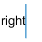'Position' - 文本位置

文本位置，指定为 [x y] 格式的二元素向量或 [x y z] 格式的三元素向量。如果省略第三个元素 z，则 MATLAB 将其设置为 0。使用数值指定位置。要将日期时间或持续时间值转换为特定坐标方向上的相应数值，可以参考ruler2num。
默认情况下，位置值是以数据单位定义的。要更改单位，使用Units属性。
例如：[0.5 0.5 0]
数据类型： double | single | int8 | int16 | int32 | int64 | uint8 | uint16 | uint32 | uint64

'Units' - 位置和范围单位

位置单位，指定为此表中的一个值。
单位说明'data'（默认值）数据坐标。'normalized'针对坐标区进行归一化。坐标区的左下角映射到 (0,0)，右上角映射到 (1,1)。'inches'英寸。'centimeters'厘米。'characters' 基于默认系统字体的字符大小。
字符宽度 = 字母 x 的宽度。  字符高度 = 两个文本行的基线之间的距离。   'points'磅。一磅等于 1/72 英寸。'pixels' 像素。 从 R2015b 开始，以像素为单位的距离不再依赖 Windows® 和 Macintosh 系统上的系统分辨率：
在 Windows 系统上，一个像素是 1/96 英寸。  在 Macintosh 系统上，一个像素是 1/72 英寸。 在 Linux® 系统上，一个像素的大小由系统分辨率确定。
所有单位（'data' 除外）都是从坐标区的左下角进行测量的。此属性会影响Position和Extent属性。
如果在创建 Text 对象时以 Name,Value 对组的形式指定 Position 和 Units 属性，则指定顺序很重要。若要使用特定单位定义位置，请在设置Position属性之前先设置 Units 属性。

'Interpreter' - 文本解释器

文本解释器，指定为下列值之一：
'tex' - 使用 TeX 标记子集解释字符。  'latex' - 使用 LaTeX 标记解释字符。  'none' - 显示字面字符。
TeX 标记
默认情况下，MATLAB 支持一部分 TeX 标记。使用 TeX 标记可添加下标和上标，修改字体类型和颜色，并在文本中包括特殊字符。
修饰符会一直作用到文本结尾，但上标和下标除外，因为它们仅修饰下一个字符或花括号中的字符。当将解释器设置为 'tex' 时，支持的修饰符如下所示。
修饰符说明示例^{ }上标'text^{superscript}'_{ }下标'text_{subscript}'\bf粗体'\bf text'\it斜体'\it text'\sl伪斜体（通常与斜体相同）'\sl text'\rm常规字体'\rm text'\fontname{specifier}字体名称 - 将 specifier 替换为字体系列的名称。您可以将此说明符与其他修饰符结合使用。'\fontname{Courier} text'\fontsize{specifier}字体大小 - 将 specifier 替换为以磅为单位的数值标量值。'\fontsize{15} text'\color{specifier}字体颜色 - 将 specifier 替换为以下颜色之一：red、green、yellow、magenta、blue、black、white、gray、darkGreen、orange 或 lightBlue。'\color{magenta} text'\color[rgb]{specifier}自定义字体颜色 - 将 specifier 替换为三元素 RGB 三元组。'\color[rgb]{0,0.5,0.5} text'
下表列出了 'tex' 解释器所支持的特殊字符。
字符序列符号字符序列符号字符序列符号 \alpha  α  \upsilon  υ  \sim  ~  \angle  ∠  \phi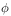\leq  ≤  \ast  *  \chi  χ  \infty  ∞  \beta  β  \psi  ψ  \clubsuit  ♣  \gamma  γ  \omega  ω  \diamondsuit  ♦  \delta  δ  \Gamma  Γ  \heartsuit  ♥  \epsilon  ϵ  \Delta  Δ  \spadesuit  ♠  \zeta  ζ  \Theta  Θ  \leftrightarrow  ↔  \eta  η  \Lambda  Λ  \leftarrow  ←  \theta  θ  \Xi  Ξ  \Leftarrow  ⇐  \vartheta  ϑ  \Pi  Π  \uparrow  ↑  \iota  ι  \Sigma  Σ  \rightarrow  →  \kappa  κ  \Upsilon  ϒ  \Rightarrow  ⇒  \lambda  λ  \Phi  Φ  \downarrow  ↓  \mu  µ  \Psi  Ψ  \circ  º  \nu  ν  \Omega  Ω  \pm  ±  \xi  ξ  \forall  ∀  \geq  ≥  \pi  π  \exists  ∃  \propto  ∝  \rho  ρ  \ni  ∍  \partial  ∂  \sigma  σ  \cong  ≅  \bullet  •  \varsigma  ς  \approx  ≈  \div  ÷  \tau  τ  \Re  ℜ  \neq  ≠  \equiv  ≡  \oplus  ⊕  \aleph  ℵ  \Im  ℑ  \cup  ∪  \wp  ℘  \otimes  ⊗  \subseteq  ⊆  \oslash  ∅  \cap  ∩  \in  ∈  \supseteq  ⊇  \supset  ⊃  \lceil  ⌈  \subset  ⊂  \int  ∫  \cdot  ·  \o  ο  \rfloor  ⌋  \neg  ¬  \nabla  ∇  \lfloor  ⌊  \times  x  \ldots  ...  \perp  ⊥  \surd  √  \prime  ´  \wedge  ∧  \varpi  ϖ  \0  ∅  \rceil  ⌉  \rangle  〉  \mid  |  \vee  ∨  \langle  〈  \copyright  ©
LaTeX 标记
要使用 LaTeX 标记，请将解释器设置为 'latex'。使用美元符号将文本括起来，例如，对于内联模式，使用 '$\int_1^{20} x^2 dx$'；对于显示模式，使用 '$$\int_1^{20} x^2 dx$$'。
显示的文本将使用默认的 LaTeX 字体样式。FontName、FontWeight 和 FontAngle 属性不起任何作用。要更改字体样式，请使用 LaTeX 标记。
用于 LaTeX 解释器的文本最多可以包含 1200 个字符。对于多行文本，请在此数量的基础上每行减少约 10 个字符。
有关 LaTeX 系统的详细信息，请访问 LaTeX Project 网站：https://www.latex-project.org/。
3.输出参数

t - Text 对象

Text 对象。在创建特定 Text 对象后，使用 t 的元素对其进行修改。有关属性列表，请参阅Text属性。

提示

默认情况下，文本对象的Clipping属性设置为 'off'，这样文本可能显示在坐标区之外。若要裁剪文本使其在坐标区边界内，请将该属性设置为 'on'。
二.说明

text(x,y,txt) 使用由 txt 指定的文本，向当前坐标区中的一个或多个数据点添加文本说明。若要将文本添加到一个点，请将 x 和 y 指定为标量。若要将文本添加到多个点，请将 x 和 y 指定为长度相同的向量。

text(x,y,z,txt) 在三维坐标中定位文本。

text(___,Name,Value)使用一个或多个名称-值对组指定 Text 对象的属性。例如，'FontSize',14 将字体大小设置为 14 磅。可以使用上述语法中的任意输入参数组合指定文本属性。如果您将 Position 和 String 属性指定为名称-值对组，则不需要指定 x、y、z 和 txt 输入。

text(ax,___) 将在由 ax 指定的笛卡尔坐标区、极坐标区或地理坐标区中创建文本，而不是在当前坐标区 (gca) 中创建文本。选项 ax 可以位于前面的语法中的任何输入参数组合之前。

t= text(___) 返回一个或多个文本对象。使用 t 修改所创建的文本对象的属性。有关属性和说明的列表，可以参考Text属性。可以使用上述任意语法指定一个输出。

三.示例
1.向数据点添加文本说明
绘制一条正弦曲线。在点 (π,0) 处，添加文本说明 sin(π)。使用 TeX 标记 \pi 表示希腊字母 π。使用 \leftarrow 显示一个向左箭头。如下所示：
x = 0:pi/20:2*pi;
y = sin(x);
plot(x,y)
text(pi,0,'\leftarrow sin(\pi)')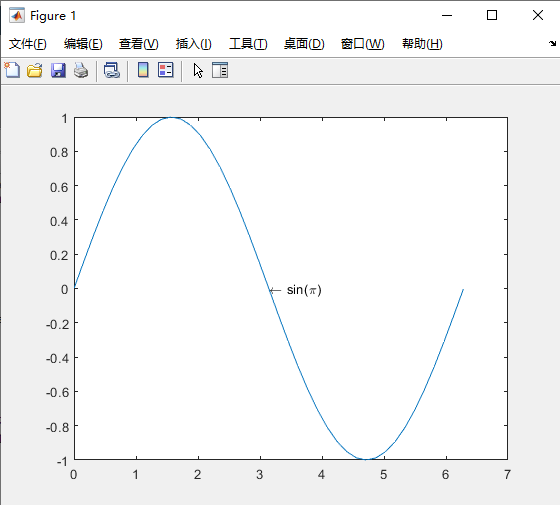有关希腊字母和其他TeX 标记的列表，请参考 Interpreter 属性说明。
2.向多个数据点添加文本
绘制一个线条。将相同的文本添加到沿该线条的两个点。如下所示：
x = linspace(-5,5);
y = x.^3-12*x;
plot(x,y)

xt = [-2 2];
yt = [16 -16];
str = 'dy/dx = 0';
text(xt,yt,str)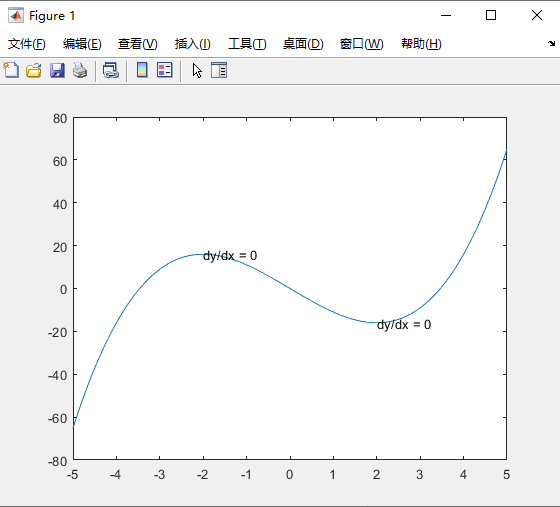通过将 str 指定为元胞数组，向每个点添加不同文本。如下所示：
x = linspace(-5,5);
y = x.^3-12*x;
plot(x,y)

xt = [-2 2];
yt = [16 -16];
str = {'local max','local min'};
text(xt,yt,str)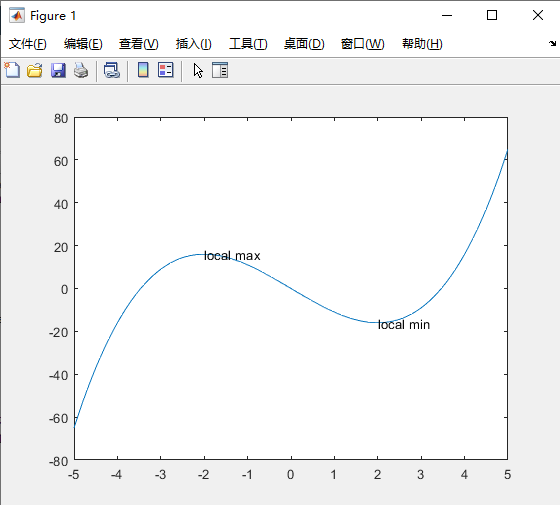3.显示多行文本
创建一个线图，并向坐标区添加一个文本说明。通过将 str 指定为元胞数组显示多行文本。如下所示：
plot(1:10)
str = {'A simple plot','from 1 to 10'};
text(2,7,str)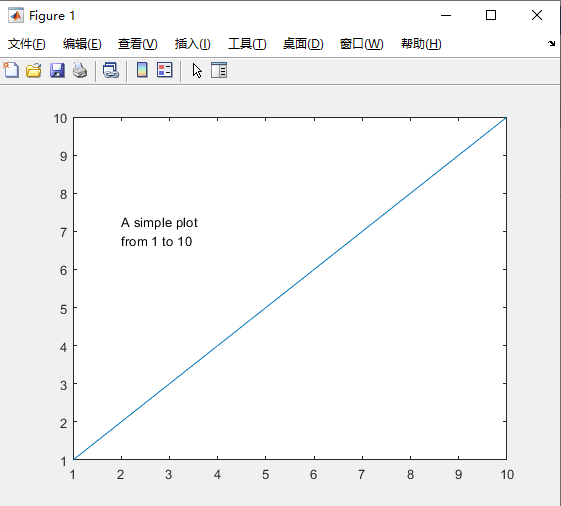创建一个线图，并向坐标区添加两个文本说明。在向坐标区添加多个文本说明时，通过指定嵌套元胞数组显示多行文本。如下所示：
plot(1:10)
str = {{'A simple plot','from 1 to 10'},'y = x'};
text([2 8],[7 7],str)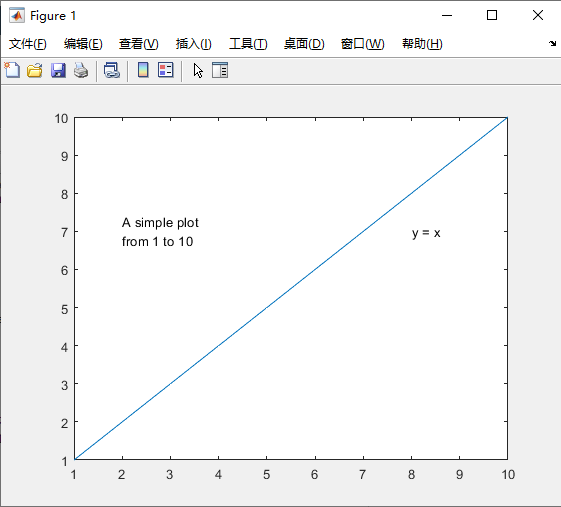4.指定文本大小和颜色
创建一个线图，并向坐标区添加文本说明。使用红色 14 号字体。如下所示：
plot(1:10)
text(2,8,'A Simple Plot','Color','red','FontSize',14)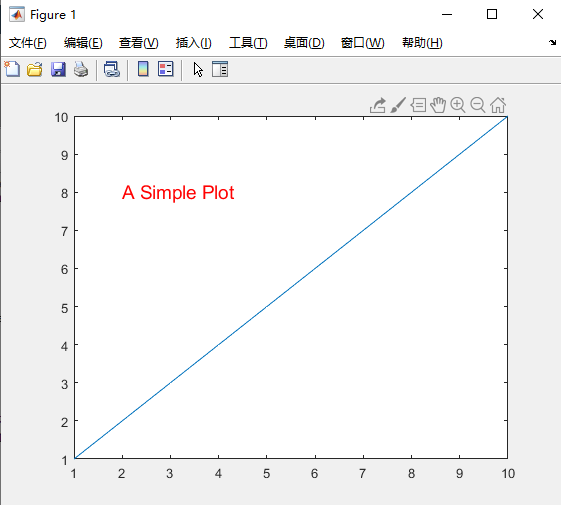5.修改现有文本
创建一个线图，并沿线条添加两个文本说明。返回文本对象 t。如下所示：
x = linspace(-5,5);
y = x.^3-12*x;
plot(x,y)
t = text([-2 2],[16 -16],'dy/dx = 0')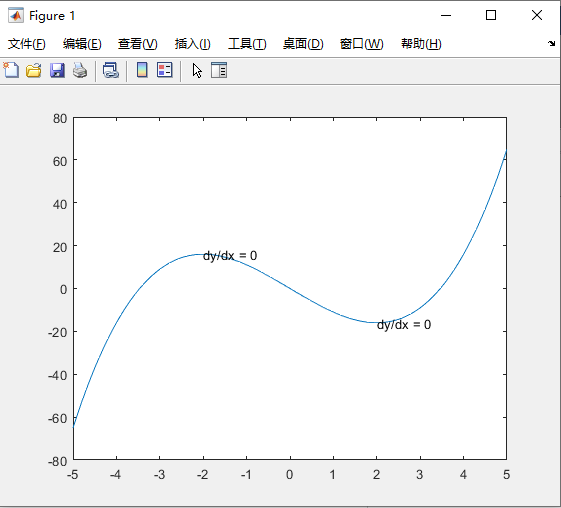t =
2x1 Text array:

Text    (dy/dx = 0)
Text    (dy/dx = 0)
text 函数为每个文本说明创建一个文本对象。因此，t 包含两个文本对象。
使用 t(1) 更改第一个文本对象的颜色和字体大小。使用圆点表示法设置属性。如果使用的是较早的版本，请改用 set 函数。
x = linspace(-5,5);
y = x.^3-12*x;
plot(x,y);
t = text([-2 2],[16 -16],'dy/dx = 0');
t(1).Color = 'red';
t(1).FontSize = 14;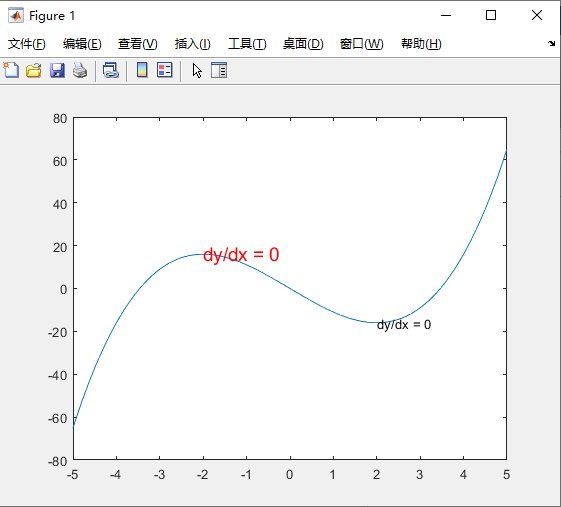展开全文• 一、text 函数、 二、annotation 函数、 三、绘制图像示例、


文章目录
一、text 函数二、annotation 函数三、绘制图像示例

一、text 函数

参考文档 : https://ww2.mathworks.cn/help/matlab/ref/text.html
使用 text 函数 , 可以在坐标系的指定位置绘制文字 ;
在 坐标系的

(

0.5

,

0.5

)

(0.5,0.5)

点位置 , 绘制积分符号文字 , 该文字是 latext 数学公式 ;

代码示例 :
% latext 数学公式 , 积分
str = '$$\int_{0}^{2} x^2\sin(x)dx$$';

% 在 0.5 0.5 位置绘制字符串
% 'Interpreter', 'latex' 表示字符串的格式是 Latex 数学公式
% 后两个参数是固定写法
text(0.5, 0.5, str, 'Interpreter', 'latex');


这里涉及到 latext 语法 :
$$\int_{0}^{2} x^2\sin(x)dx$$

该 latext 数学公式展示效果如下 :

∫

0

2

x

2

sin

⁡

(

x

)

d

x

\int_{0}^{2} x^2\sin(x)dx

运行结果 :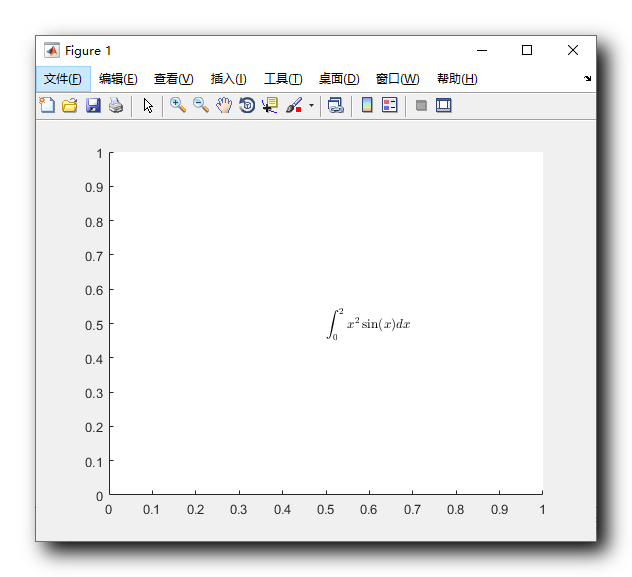二、annotation 函数

参考文档 : https://ww2.mathworks.cn/help/matlab/ref/annotation.html

使用 annotation 函数绘制箭头 :
% latext 数学公式 , 积分
str = '$$\int_{0}^{2} x^2\sin(x)dx$$';

% 在 0.5 0.5 位置绘制字符串
% 'Interpreter', 'latex' 表示字符串的格式是 Latex 数学公式
% 后两个参数是固定写法
text(0.5, 0.5, str, 'Interpreter', 'latex');

% 绘制一个箭头 ,
% 'X', [0.2, 0.5], 表示 x 轴方向 , 从 0.2 到 0.5
% 'Y', [0.2, 0.5], 表示 y 轴方向 ,, 从 0.2 到 0.5
annotation('arrow', 'X', [0.2, 0.5], 'Y', [0.2, 0.5] );

运行效果 :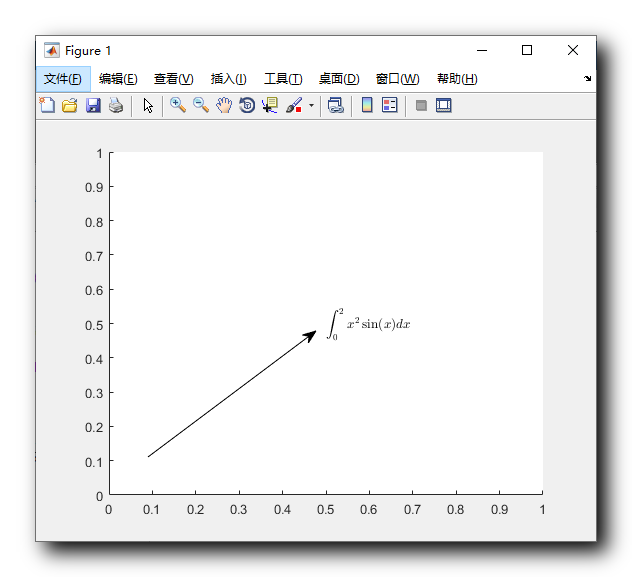三、绘制图像示例

绘制两个图像 :

f

=

t

2

f = t^2

g

=

s

i

n

(

2

π

t

)

g = sin(2 \pi t)

绘图范围是

[

1

,

2

]

[1,2]

之间 ;

代码 :
% x 轴变量
t = 0 : 0.05 : 2;
% 函数1 平方函数
f = power(t, 2);
% 函数2 正弦函数
g = sin(2 * pi * t);

% 绘制多个图像
hold on

% 绘制平方函数
% 实线 + 红色 + 圆
plot(t, f, 'or-');
% 绘制正弦函数
% 虚线 + 绿色 + 三角
plot(t, g, '^g--');

% 第一个曲线设置 f=t^2
% 第二个曲线设置 g=sin(2\pi t)
% 设置位置 , 左上角 , 'Location','northwest'
legend('f=t^2', 'g=sin(2\pi t)', 'Location','northwest');

% y 轴标签
ylabel('f(t)');
% x 轴标签
xlabel('时间 (ms)');

% 标题
title('时间函数')

% 绘制多个图像
hold off


执行结果 :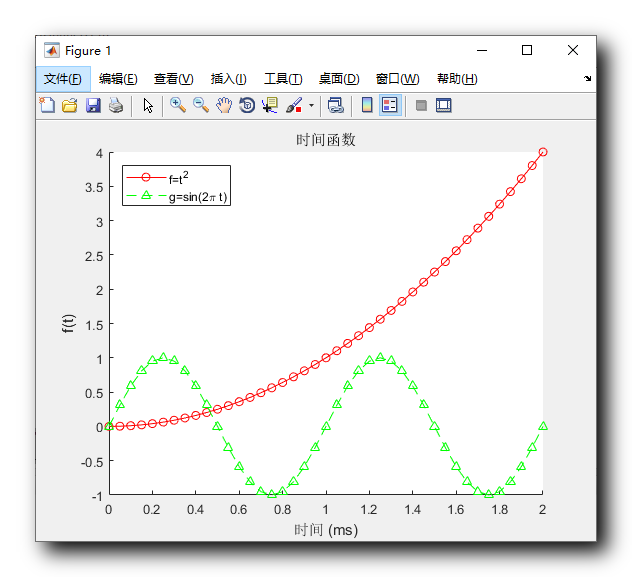展开全文annotation
• 本代码主要利用MATLAB工具实现MATLAB——在绘图窗口创建text对象，简单明了，易于理解
• Matlab作图实例——xlabel,ylabel,title,text,plot,patch,datetime等的应用做线性图，并用变量标记每个点做US inflation相关的图test 绘制区间阴影test 绘制美丽的柱状图新浪微博 做线性图，并用变量标记每个点 ...
• 实验三 MATLAB 绘图 一实验目的 1掌握二维图形的绘制 2掌握图形的标注 3了解三维曲线和曲面图形的绘制 二实验的设备及条件 计算机一台带有 MATLAB7.0 以上的软件环境 设计提示 1Matlab 允许在一个图形中画多条曲线...
• ## Matlab绘图基础

千次阅读 2019-01-22 22:02:21
Matlab绘图基础（一） plot()函数 plot(x, y)：画每一个点(x, y) plot(y)：画出每一个点(x, y)，x是[1……n]，n = length(y)，x默认依次增加1 Example01：plot(cos(0:pi/20:2pi))； Example02：（…三个点表示...
• MATLAB中绘制图形，通常采用以下7个步骤 1.准备数据 准备好绘图需要的横坐标变量和纵坐标变量数据。 2.设置当前绘图区 在指定的位置创建新的绘图窗口，并自动以此窗口的绘图为当前绘图区。 3.绘制图形 创建坐标轴...
• ## MATLAB绘图总结

千次阅读 多人点赞 2021-01-31 18:56:03
目录一些常用的MATLAB绘图方法二维图像绘制三维作图 提示：文章写完后，目录可以自动生成，如何生成可参考右边的帮助文档 一些常用的MATLAB绘图方法 总结一下一些常用的MATLAB的绘图函数和参数设置，增强结果的可视...数学建模
• text对象 （1）text(x坐标，y坐标，'string')在图形中指定位置（x，y）显示字符串string。（2）Editing有效值为on/off，off时，用户在执行GUI操作时无法直接在该Text对象上进行编辑，改为on后，可以进行编辑。（3）...
• matlab读取txt文件并作图，txt为光谱仪测量的反射率数据，附上代码和反射率数据。
• ## Matlab绘图

千次阅读 2018-05-27 10:36:24
Matlab绘图 强大的绘图功能是Matlab的特点之一，Matlab提供了一系列的绘图函数，用户不需要过多的考虑绘图的细节，只需要给出一些基本参数就能得到所需图形，这类函数称为高层绘图函数。此外，Matlab还提供了直接对...
• MATLAB绘图 最大化全屏后保存一、绘图[^1]二、图例[^2]添加图例的方式一：添加图例的方式二设置图例的排列方式图例的位置和列数显示指定曲线的图例图例背景和轮廓三、保存[^3]参考资料欢迎使用Markdown编辑器新的...
• ## Matlab绘图方法整理(超完整版)

万次阅读 多人点赞 2020-02-22 10:48:32
超详细版Matlab绘图方法整理，1万字文章让你流畅掌握Matlab作图方法，保证你能完全掌握常用作图手段，谁又不想做一幅详细、美丽的图呢？数学建模 数据可视化
• plot（x,y,‘str’）绘图的基础图像更改 其中字符所代表的意思如下图 举个例子 hold on plot(cos(0:pi/20:2 pi),‘o–b’) ; plot(sin(0:pi/20:2 pi),‘x-y’) ; hold off 只需将所需要的形式...
• 之前用MATLAB绘图都是正常的光滑图线，可能是前天算一个程序一直算不出来我强制退出了，后面再用MATLAB绘图就出现锯齿，而且加了'linesmootjing''on'也没用！ 求助大家有...开发语言 有问必答
• text(-10,-10,fid);%文本框左下角坐标 Frame = getframe(fig);%获取frame Img = frame2im(Frame);%将frame转换成imwrite函数可以识别的格式 imwrite(Img,'test.jpg')%保存到当前目录下，图片名字为test.jpg 实例2：...plot 坐标
• 1.绘图语句大全 2.轴属性设置常用函数 2.1set与get函数 2.2坐标轴设置 2.3其他 1.绘图语句大全 函数名称 功能 plot 绘制线状图 scatter 绘制散点图 bar 绘制二维条状图 barh 绘制二维...
• ## 【Matlab】MATLAB绘图

万次阅读 多人点赞 2018-06-29 17:18:39
专题四 MATLAB绘图 绘图的目的是使数据可视化。 一 二维曲线 1. 函数plot() 在MATLAB中，函数plot()是最基本的绘图函数，利用它可以绘制出不同的二维曲线。函数plot()的基本用法： plot(x, y) % 其中，x和y...
• ## matlab绘图命令汇总

万次阅读 多人点赞 2018-07-06 16:11:40
MATLAB绘图虽说非常简单，但是众多命令不好记忆，每次用到都要到网上查找也非常麻烦，找到一个比较全的汇总，以后用到的时候直接来这里就行了。a=linspace(1,2,10)plot(a,'--pr','linewidth',1.5,'MarkerEdgeColor',...
• 含选项的plot函数 Matlab提供了一些绘图选项，用于确定所绘曲线的线型、颜色和数据点标记符号。这些选项如表所示： 例: 用不同的线型和颜色在同一坐标内绘制曲线 及其包络线。 x=(0:pi/100:2*pi)'; y1=2*exp(-0.5*x...数学建模
• 有同学说，靠网络上的文章，很难...本期推文，喵姐会介绍一下matlab作图，尽量让各位同学通过一篇推文学会matlab作图从入门到熟练。如果你对matlab已经有所了解，可以跳过下面的入门部分。思想部分为什么我们要用MAT...
• grid（axes_handle,on|off）: 对指定的坐标轴axes_handle是否显示分割线，这主要是使用在三维的绘图中 如下面的例子所示： x=linspace(0,2*pi,100); >> y1=sin(x); >> y2=cos(x); >> h=plot(x,y1,'-r',x,y2,'...
• MATLAB学习笔记4 MATLAB绘图 文章目录MATLAB学习笔记4 MATLAB绘图4.1 二维曲线plot函数fplot函数4.2 绘制图形的辅助操作图形标注坐标控制图形保持图形窗口的分割4.3 其他形式的二维图形对数坐标图极坐标图统计图4.4 ...
• Matlab绘图强大的绘图功能是Matlab的特点之一，Matlab提供了一系列的绘图函数，用户不需要过多的考虑绘图的细节，只需要给出一些基本参数就能得到所需图形，这类函数称为高层绘图函数。此外，Matlab还提供了直接对...
• ## matlab绘图设置 （全）

万次阅读 多人点赞 2018-03-25 19:12:52
2) 坐标轴控制:MATLAB的缺省方式是在绘图时,将所在的坐标系也画出来,为隐去坐标系,可用axis off;axis on则显示坐标轴3)通常MATLAB的坐标系是长方形,长宽比例大约是 4:3,为了得到一个正方形的坐标系可用:...
• 本篇文章非完全原创，我的工作就是把见到的Matlab绘图代码收集起来重新跑一遍，修改局部错误，然后将所有的图贴上来供大家参考。大家可以先看图，有看中的可以直接把代码Copy过去改成自己想要的。 <br
• ## Matlab绘图基本用法

千次阅读 2020-05-21 21:02:30
• ## Matlab绘图-详细全面（图）

万次阅读 多人点赞 2016-07-10 21:16:08
全面Matlab基础绘图知识。强大的绘图功能是Matlab的特点之一，Matlab提供了一系列的绘图函数，用户不需要过多的考虑绘图的细节，只需要给出一些基本参数就能得到所需图形，这类函数称为高层绘图函数...

# matlab绘图textmatlab 订阅